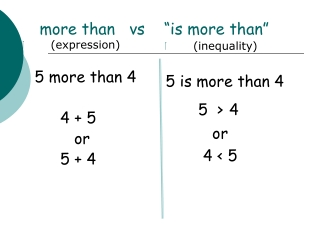DownloadDownload Presentationmore than vs “is more than”

# more than vs “is more than”

Download Presentation## more than vs “is more than”

- - - - - - - - - - - - - - - - - - - - - - - - - - - E N D - - - - - - - - - - - - - - - - - - - - - - - - - - -
##### Presentation Transcript

1. (expression) 5 more than 4 4 + 5 or 5 + 4 (inequality) 5 is more than 4 5 >4 or 4 < 5 more than vs “is more than”

2. (expression) 2 less than 8 (inequality) 2 “is” less than 8 2 < 8 or 8 > 2 less than vs “is less than” 8 - 2 Has to be written in the order above! Subtraction is not commutative . The phrase “less than” changes the order of the numbers.

3. Consecutive ODD integers 1,3,5,7,9,11,13,15… Consecutive EVEN integers 2,4,6,8,10,12,14… The Integers…-3,-2,-1,0,1,2,3… “consecutive”means following each other in uninterrupted order

4. 1.The sum of three consecutive integers is 12. Write this relationship as an equation. 3,4,5 2. The length of the sides of a triangle are consecutive even integers and the perimeter is 36. Write an equation. 10,12,14 X+(X +1)+(X+2) = 12 2. X+(x+2)+(X+4) = 36 Exercises

5. A little more translating • 6 less than x is at most 20 x-6 ≤ 20 (inequality) • The sum of the two consecutive odd integers is more than 10 x+(x+2) > 10 (inequality) • 5 more than ½ of a number is 3 5 + 1/2x = 3 (equation)

6. The absolute value of -6 is the opposite of -6 l -6 l = 6 All real numbers greater than 2 is at most 7 2 < x ≤ 7 Ten less than two times the sum of x and 4 2 (x+4) - 10